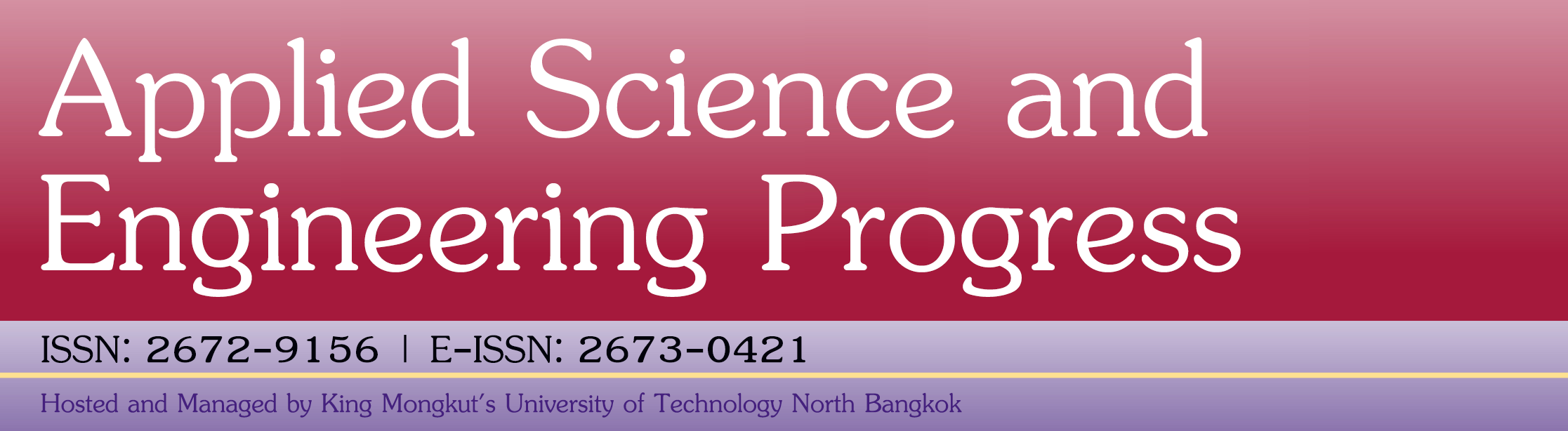#### On the Average Run Lengths of Quality Control Schemes Using a Numerical Integral Equation Approach

Piyatida Saesuntia, Yupaporn Areepong, Saowanit Sukparungsee

#### Abstract

This research presents the approach of estimating the average run length (ARL) by using the numerical integral equation (NIE) approach, such as the Gaussian, Midpoint, Trapezoidal, and Simpson’s rules for the extended exponentially weighted moving average (EEWMA) control chart, when observations are continuous distributions namely exponential, Weibull and Gamma distributions. In addition, the performance of the extended exponentially weighted moving average (EEWMA) control chart is compared with the modified exponentially weighted moving average (modified EWMA) and exponentially weighted moving average (EWMA) control charts. The performance metric is the out-of-control average run length (ARL1). The results show that the EEWMA control chart performs the best among the modified EWMA and EWMA control charts. Furthermore, the efficacies of the control charts using the approximated ARL solutions were also applied to real-world applications.

#### Keywords

Full Text: PDF

DOI: 10.14416/j.asep.2022.05.002

### Refbacks

• There are currently no refbacks.